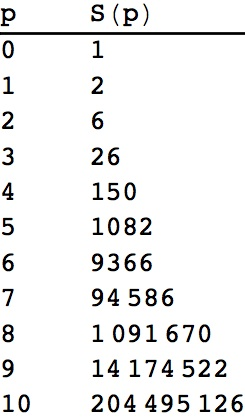# Questions about some infinite series and necklaces of partitions of labeled beads

Posted by: Gary Ernest Davis on: November 5, 2013

We learn early in a study of infinite series that the geometric series$S=\sum_{k=1}^{\infty}1/2^k$ sums to 1.

Sometimes you will see this sort of reasoning:$S=1/2+1/4+1/8+ \ldots$ so$2S=1+(1+1/2+1/4+\ldots =1+S$ so$S=1$

which is somewhat suspect in light of Euler’s “argument”:$1+2+4+8+16+\ldots=1+2(1+2+4+8+\ldots)$ soÂ$1+2+4+8+16+\ldots = -1$.

We need first to know thatÂ$S=\sum_{k=1}^{\infty}1/2^k$ converges absolutely, which we can do, for example by a use of the ratio test:

the$(k+1)^{\textrm{th}}$ term ofÂ$S=\sum_{k=1}^{\infty}1/2^k$ divided by the$k^{\textrm{th}}$ term is 1/2 <1.

Another application of the ratio test shows that theÂ series$\sum_{k=1}^{\infty}k/2^k$ is absolutely convergent:

theÂ$(k+1)^{\textrm{th}}$ of this series is$(k+1)/2^{k+1}$, the$k^{\textrm{th}}$ term isÂ$k/2^k$ and their ratio isÂ$\frac{(k+1)}{2^{k+1}}\frac{2^k}{k}=\frac{1}{2}(1+1/k)$ which approaches$1/2$ as$k \to \infty$.

If we denoteÂ$T=\sum_{k=1}^{\infty}k/2^k$ then a simple, and legitimate, calculation shows that$2T-T=1+1/2+1/4+1/8+\ldots$which we Â know to be$1+1=2$.

In fact, for any natural number$p$ the ratio test shows that the series$S(p):=\sum_{k=1}^{\infty}k^p/2^k$ is absolutely convergent.

A MathematicaÂ® calculation:

TableForm[Table[{p, Limit[Sum[k^p/2^k, {k, 1, n}], n -> Infinity]}, {p, 0, 10}], TableHeadings -> {None, {“p”, “S(p)”}}]

Â yields the following results for$S(p)$:The first curious thing is that the results are all whole numbers. Why is that?

The second curious thing is that if we enter this sequence of whole numbers into theÂ The On-Line Encyclopedia of Integer SequencesÂ we get a match:

these whole numbers match the number of necklaces of partitions of p+1 labeled beads. They also match the sequence of cumulants of the probability distribution of the number of tails before the first head in a sequence of fair coin tosses. Is that right? If so, why?Â$p=2$ is the first really interesting case.

A detailed account of these and similar series is dealt with byÂ MirceaÂ CÃ®rnu “DeterminantalÂ formulas forÂ sumÂ of generalizedÂ arithmetic-geometric series“Â Boletn de la Asociacion Matematica Venezolana, Vol. XVIII, No. 1 (2011), 13-25.

Enjoy! It’s connections like these that give mathematicians a buzz.